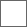• 10回复贴，共1

### 给做整合同学的教程-全屏特效自适应分辨率的写法

[Statedef 40001];02UM大背景
anim = 9999
。。。

[State 40001, Tile #1-7]
type = Explod
triggerall = !numexplod(15600)
trigger1 = !time
anim = ifelse((root,var(58) = 0),15600,15610)
ID = 15600
pos = 0,0
postype = left ;p2,front,back,left,right
removetime = 10
pausemovetime = -1
supermovetime = -1
scale = 1,(4*gameheight) / (gamewidth*3)
;此处普屏 4:3时 scale为1:1
; 宽屏 16:9时 scale需调整为1:.75
;当人物localcoord = 320,180(或240也可)时
;由于mugen可读取mugen.cfg中gameheight，gamewidth数据(不写默认240:320)
;代入后比例即为自适应
sprpriority = -20
ownpal = 1
ignorehitpause = 1
bindtime = -1
persistent = 0

;坐标pos和大小scale都应按比例补正
[State 40001, P2 BG];如红蓝长条
type = Explod
triggerall = !numexplod(15651)
;triggerall = palno = [1,6]
triggerall = teamside = 2
trigger1 = !time
anim = 10010
ID = 15651
pos = 0,abs((240*var(0))-81)*(4*gameheight) / (gamewidth*3)
postype = right ;p2,front,back,left,right
pausemovetime = -1
supermovetime = -1
removetime = -2
bindtime = -1
scale = 1,(4*gameheight) / (gamewidth*3) ;0.75
facing = -1
sprpriority = -20
ownpal = 1
ignorehitpause = 1

[State 40001, P1 PORTRAIT];UM头像
type = Explod
triggerall = !numexplod(15660)
triggerall = teamside = 1
trigger1 = !time
anim = ifelse((root,var(58) = 0),10015,10025)
ID = 15660
pos = 0,abs((240*var(0))-81)*(4*gameheight) / (gamewidth*3)
postype = left ;p2,front,back,left,right
pausemovetime = -1
supermovetime = -1
removetime = -2
bindtime = -1
scale = 1,(4*gameheight) / (gamewidth*3) ;0.75
sprpriority = -19
ownpal = 1
ignorehitpause = 1

[State 40003, ModifyExplod];变化中也应补正
type = ModifyExplod
trigger1 = time = 2
ID = 15650
scale = 1,0.3*(4*gameheight) / (gamewidth*3) ;0.225

PS:三神器疯麻子的mugen legacy全适配通用火花版和mugen match记分系统通用火花版已改好，年前放出#### 扫二维码下载贴吧客户端